Formulae based

Chapter 2 Class 12 Inverse Trigonometric Functions
Concept wise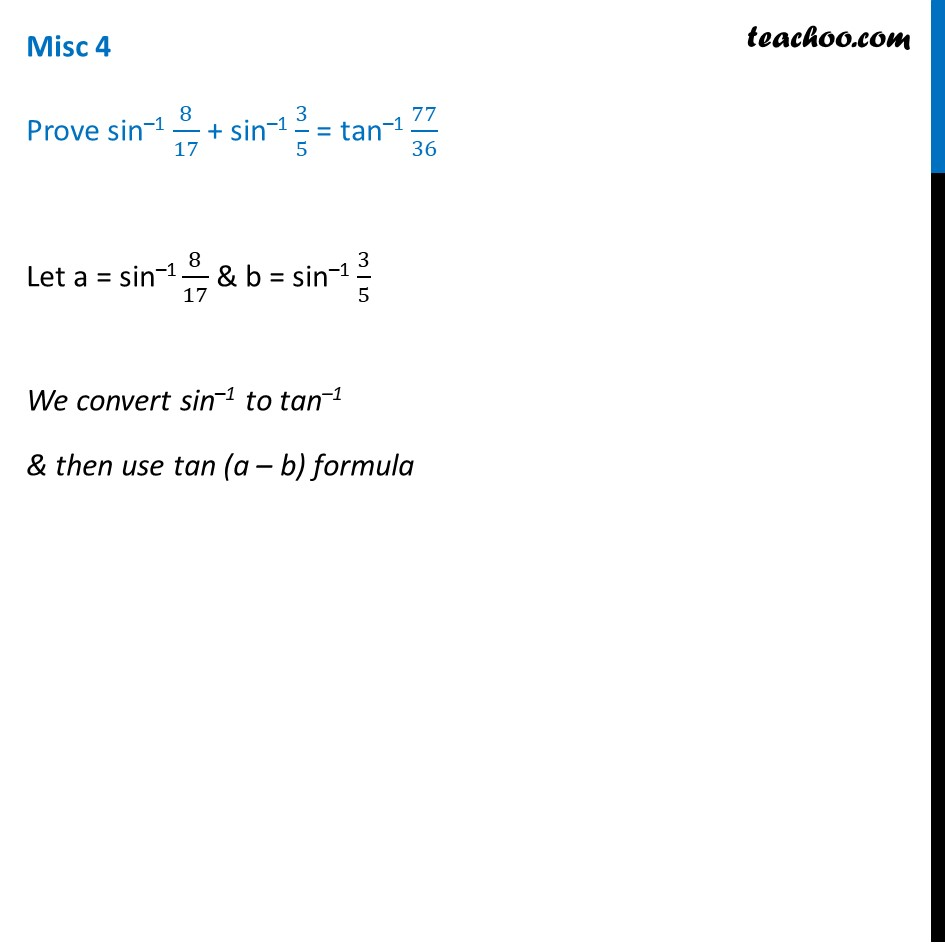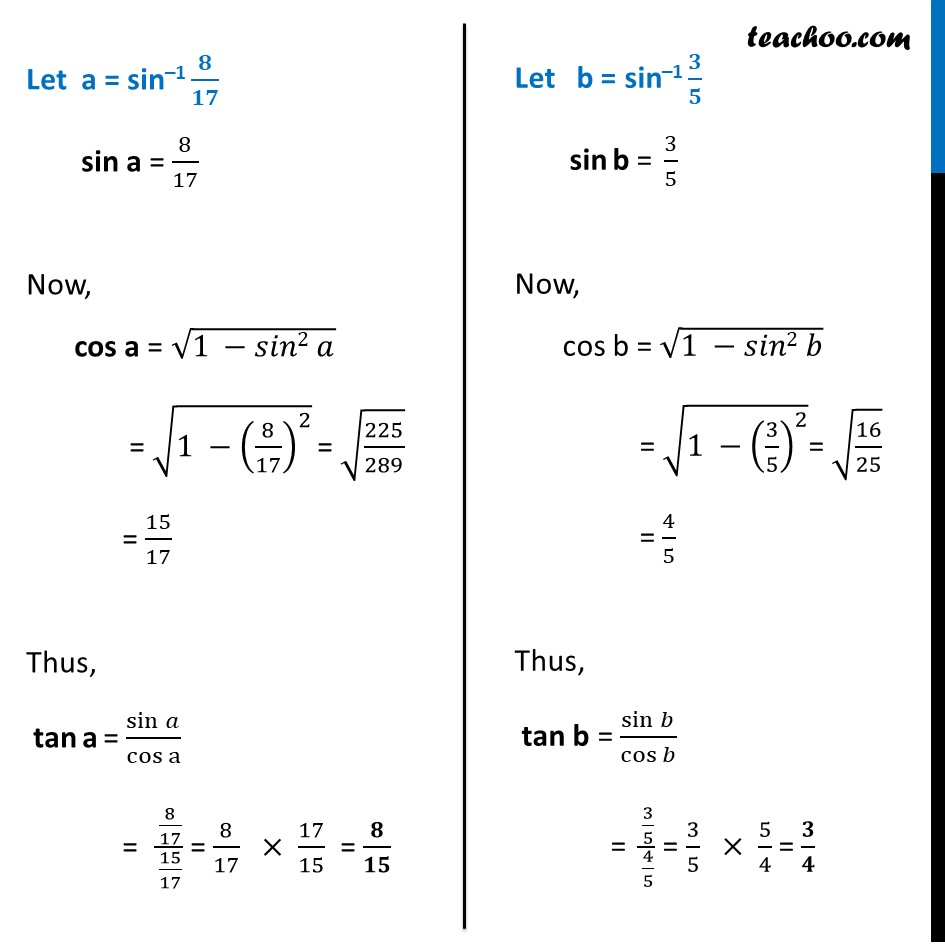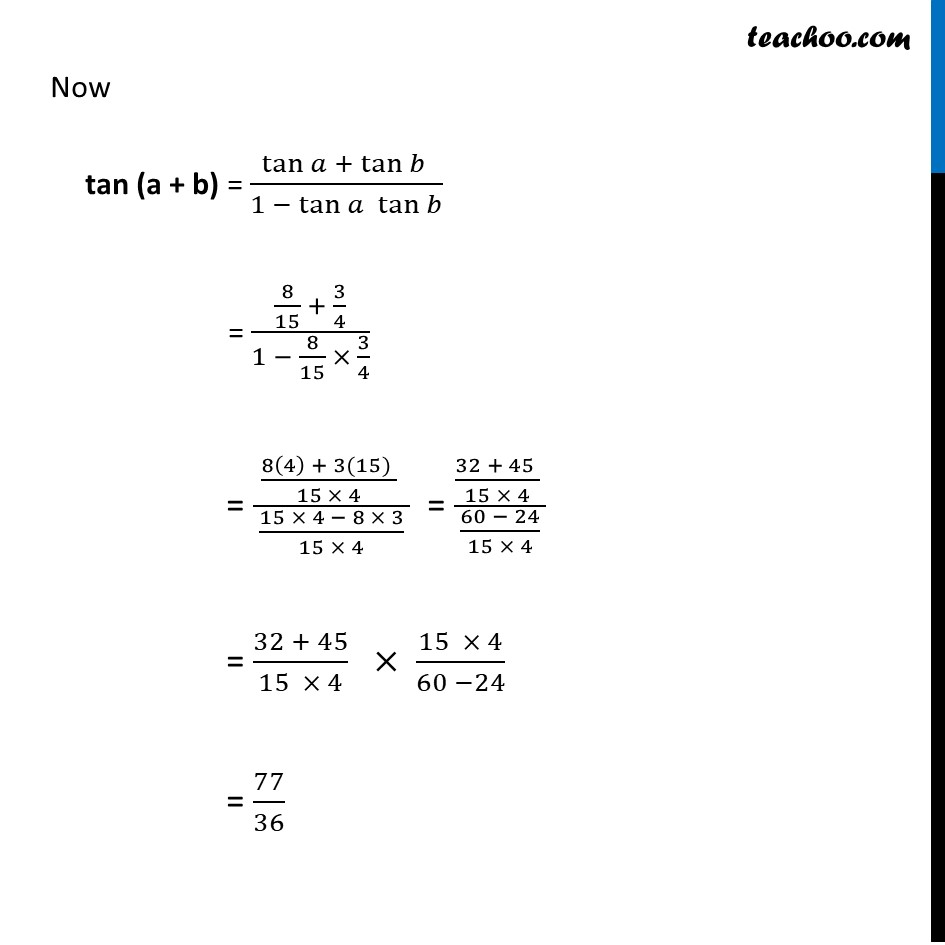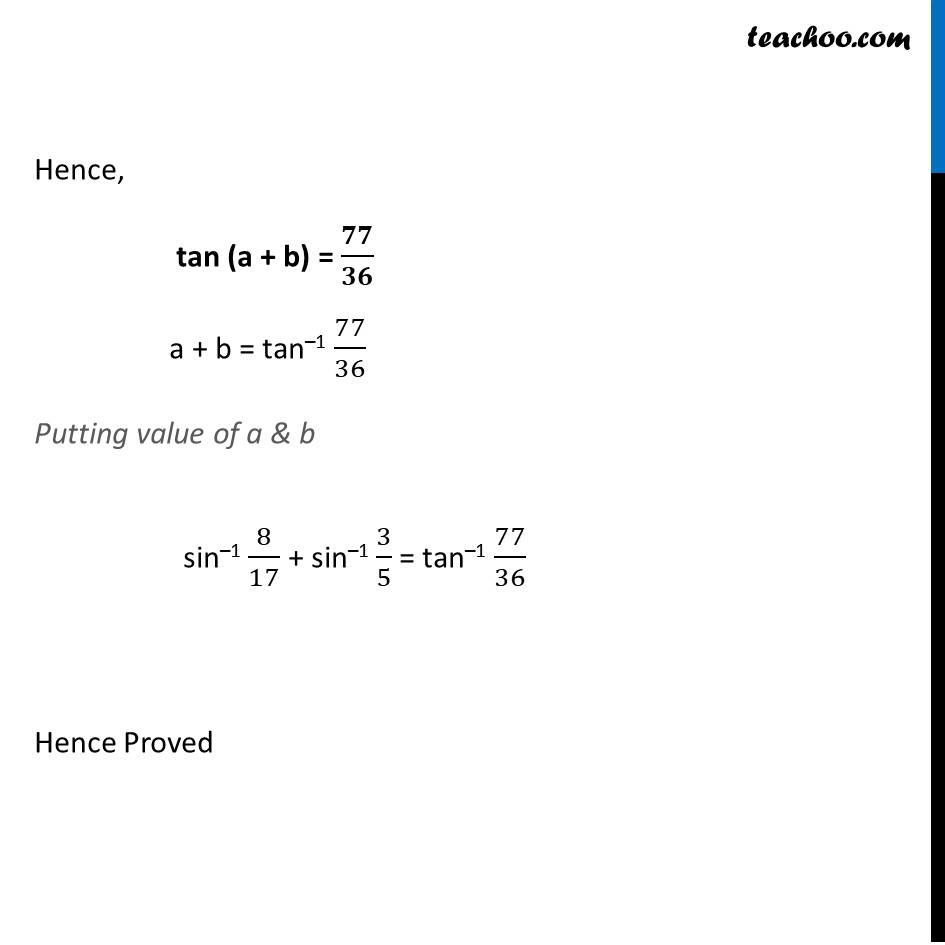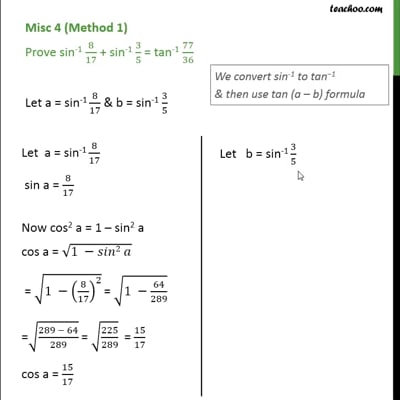This video is only available for Teachoo black users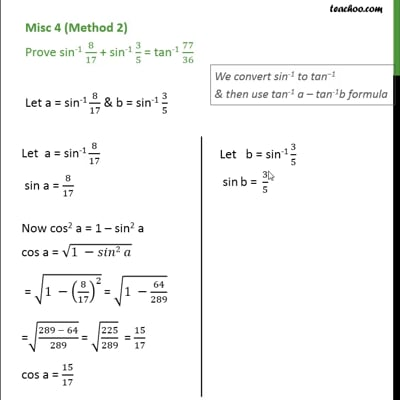This video is only available for Teachoo black users

Solve all your doubts with Teachoo Black (new monthly pack available now!)

### Transcript

Misc 4 Prove sin–1 8/17 + sin–1 3/5 = tan–1 77/36 Let a = sin–1 8/17 & b = sin–1 3/5 We convert sin–1 to tan–1 & then use tan (a – b) formula Let a = sin–1 𝟖/𝟏𝟕 sin a = 8/17 Now, cos a = √(1 −𝑠𝑖𝑛2 𝑎) = √(1 −(8/17)^2 ) = √(225/289) = 15/17 Thus, tan a = (sin 𝑎)/(cos a) = (8/17)/(15/17) = 8/17 × 17/15 = 𝟖/𝟏𝟓 Let b = sin–1 𝟑/𝟓 sin b = 3/5 Now, cos b = √(1 −𝑠𝑖𝑛2 𝑏) = √(1 −(3/5)^2 )= √(16/25) = 4/5 Thus, tan b = sin⁡𝑏/cos⁡𝑏 = (3/5)/(4/5) = 3/5 × 5/4 = 𝟑/𝟒 Now tan (a + b) = tan⁡〖𝑎 +〖 tan〗⁡〖𝑏 〗 〗/(1 − tan⁡〖𝑎 tan⁡𝑏 〗 ) = (8/15 + 3/4)/(1 − 8/15 × 3/4) = ((8(4) + 3(15) )/(15 × 4) )/( (15 × 4 − 8 × 3)/(15 × 4) ) = ((32 + 45 )/(15 × 4) )/( (60 − 24)/(15 × 4) ) = (32 + 45)/(15 × 4) × (15 × 4)/(60 −24) = 77/36 Hence, tan (a + b) = 𝟕𝟕/𝟑𝟔 a + b = tan–1 77/36 Putting value of a & b sin–1 8/17 + sin–1 3/5 = tan–1 77/36 Hence Proved